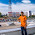## Thursday, February 18, 2010

### Option Pricing Black-Scholes script for MATLAB and FreeMAT

```function [C, P]= option_bs(S,X,r,sigma,days)
%
%  This function to calculate Call and Put price per Black-Scholes formula.
%  This function can be run both at FreeMAT and MATLAB,
%  This function should be same as MATLAB's financial toolbox function
%  blsprice.
%
% C: call price
% P: put price
% S: stock price at time 0
% X:  strike price
% r: risk-free interest rate
% sigma: volatility of the stock price measured as annual standard deviation
% days: numbers of days remaining in the option contract, this will be converted into unit of years.
% Black-Scholes formula:
%  C = S N(d1) - Xe-rt N(d2)
%  P = Xe-rt N(-d2) - S N(-d1)
%

T=days/365;

% for call
d1 = (log(S/X) + (r + 0.5*sigma^2)*T)/(sigma*sqrt(T));
d2 = d1 - sigma*sqrt(T);
N1 = 0.5*(1+erf(d1/sqrt(2)));
N2 = 0.5*(1+erf(d2/sqrt(2)));

C = S*N1-X*exp(-r*T)*N2;

% for put

N1 = 0.5*(1+erf(-d1/sqrt(2)));
N2 = 0.5*(1+erf(-d2/sqrt(2)));

P = X*exp(-r*T)*N2 - S*N1;
end
```

1.This comment has been removed by the author.

2.Thank you please send me request at rmurthyiitk@gmail.com

3.If there is n=365 how the code is for each i? (i=1,2,3,...,365)

4.Higher bonuses : Some digital options broker supply to 25% bonuses for their traders that also rely upon total turnover and initial deposit amount in the traders. Binary Options Look for such trading platforms that provide higher bonuses to their own users.